NEET  >  Test: Previous Year Questions: Chemical Equilibrium

# Test: Previous Year Questions: Chemical Equilibrium

Test Description

## 17 Questions MCQ Test Chemistry Class 11 | Test: Previous Year Questions: Chemical Equilibrium

Test: Previous Year Questions: Chemical Equilibrium for NEET 2023 is part of Chemistry Class 11 preparation. The Test: Previous Year Questions: Chemical Equilibrium questions and answers have been prepared according to the NEET exam syllabus.The Test: Previous Year Questions: Chemical Equilibrium MCQs are made for NEET 2023 Exam. Find important definitions, questions, notes, meanings, examples, exercises, MCQs and online tests for Test: Previous Year Questions: Chemical Equilibrium below.
Solutions of Test: Previous Year Questions: Chemical Equilibrium questions in English are available as part of our Chemistry Class 11 for NEET & Test: Previous Year Questions: Chemical Equilibrium solutions in Hindi for Chemistry Class 11 course. Download more important topics, notes, lectures and mock test series for NEET Exam by signing up for free. Attempt Test: Previous Year Questions: Chemical Equilibrium | 17 questions in 25 minutes | Mock test for NEET preparation | Free important questions MCQ to study Chemistry Class 11 for NEET Exam | Download free PDF with solutions
 1 Crore+ students have signed up on EduRev. Have you?
Test: Previous Year Questions: Chemical Equilibrium - Question 1

### For the reaction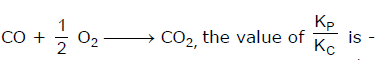[AIEEE-2002]

Detailed Solution for Test: Previous Year Questions: Chemical Equilibrium - Question 1

The correct answer is option C
Kp = Kc (RT)Δn;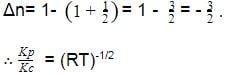Test: Previous Year Questions: Chemical Equilibrium - Question 2

### Which of the following equilibria is not affected by change in volume of the flask [AIEEE-2002]

Detailed Solution for Test: Previous Year Questions: Chemical Equilibrium - Question 2

Change in volume affects number of moles per unit volume and move in the direction which undo the change.

N2(g)+ O2(g) ⇄2NO(g)

Number of moles of reactants and products are equal.

Test: Previous Year Questions: Chemical Equilibrium - Question 3

### For the reaction equilibrium N2O4 (g) ⇌ 2NO2 (g) the concentrations of N2O4 and NO2 at equilibrium are 4.8 × 10-2 and 1.2 × 10-2 mol L-1 respectively. The value of KC for the reaction is -        [AIEEE-2003]

Detailed Solution for Test: Previous Year Questions: Chemical Equilibrium - Question 3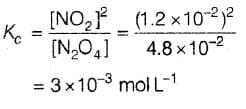Test: Previous Year Questions: Chemical Equilibrium - Question 4

Consider the reaction equilibrium  2SO2(g)+O2(g)2 SO3 (g); ΔHº = -198 kJ

On the basis of Le Chatelier's principle, the condition favourable for the forward reaction is -

[AIEEE-2003]

Detailed Solution for Test: Previous Year Questions: Chemical Equilibrium - Question 4

The correct answer is option A

ACCORDING TO LE CHATELIER'S PRINCIPLE

TEMPERATURE
if we increase temperature equilibrium will shift that direction which proceeds an endothermic reaction but the reaction given in question is exothermic so we have to decrease temperature to proceed forward.

PRESSURE
at equilibrium if we increase pressure equilibrium will shift that direction which has less number of moles but in given reaction products have less no. of moles than reactant that's why we increase pressure.

Test: Previous Year Questions: Chemical Equilibrium - Question 5

What is the equilibrium expression for the reaction P4(S) + 5O2(g)  P4O10(s) ?

[AIEEE-2004]

Detailed Solution for Test: Previous Year Questions: Chemical Equilibrium - Question 5

In the expression for equilibrium constant (Kp or Kc) species  state are not written (i.e., their molar concentrations are not taken as 1)
P4 (s) + 5O2 (g) ⇌ P4O10 (s)
Thus, Kc = 1/[O2]5

Test: Previous Year Questions: Chemical Equilibrium - Question 6

For the reaction CO(g) + Cl2(g) COCl2(g) the KP/KC is equal to -

[AIEEE-2004]

Detailed Solution for Test: Previous Year Questions: Chemical Equilibrium - Question 6

Kp =Kc (RT)Δn
Δn = sum of coefficients of gaseous products
Sum of coefficients of gaseous reactants.
CO (g) + Cl2 (g) → COCl2 (g)
Δn = 1-2 = -1
Kp = Kc (RT)-1
Kp/Kc = (RT)-1
1/RT

Test: Previous Year Questions: Chemical Equilibrium - Question 7

The equilibrium constant for the reaction N2(g) + O2(g) 2NO(g) at temperature T is 4×10-4. The value of KC for the reaction NO(g)  1/2 N2(g) + O2(g) at the same temperature is-

[AIEEE-2004]

Detailed Solution for Test: Previous Year Questions: Chemical Equilibrium - Question 7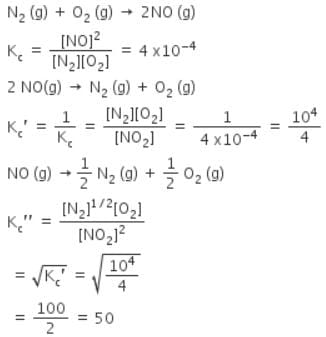Test: Previous Year Questions: Chemical Equilibrium - Question 8

For the reaction 2 NO2(g) 2 NO(g) + O2(g),

(Kc = 1.8 × 10_6 at 184ºC)

(R = 0.0831 kJ/(mol.K))

When Kp and Kc are compared at 184ºC it is found that

[AIEEE-2005]

Detailed Solution for Test: Previous Year Questions: Chemical Equilibrium - Question 8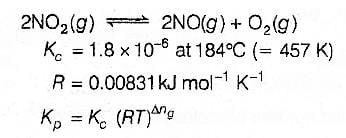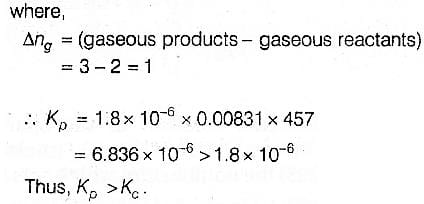Test: Previous Year Questions: Chemical Equilibrium - Question 9

The exothermic formaton of ClF3 is represented by the equation -

Cl2(g)+3F2(g) 2ClF3(g) ; ΔrH = -329 kJ

Which of the following will increase the quantity of ClF3 in an equilibrium mixture of Cl2, F2 and ClF3 ?

Detailed Solution for Test: Previous Year Questions: Chemical Equilibrium - Question 9

The correct answer is option C
According to Le-Chatelier's Principle, if a system at equilibrium is subjected to a change of concentration pressure or temperature then the equilibrium is shifted in such a way as to nullify the effect of change.
In the given reaction, if the concentration of F2 is increased then the reaction will shift in the forward direction in order to increase the concentration of ClF3.
Hence,

Test: Previous Year Questions: Chemical Equilibrium - Question 10

A schematic plot of ln Keq versus inverse of temperature for a reaction is shown below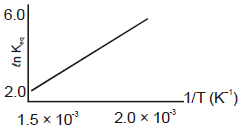The reaction must be

Detailed Solution for Test: Previous Year Questions: Chemical Equilibrium - Question 10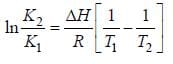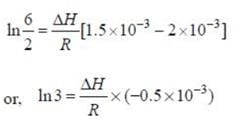ΔH Of reaction comes out to be negative. Hence reaction is exothermic.

Test: Previous Year Questions: Chemical Equilibrium - Question 11

An amount of solid NH4HS is placed in a flask already containing ammonia gas at a certain temperature and 0.50 atm pressure. Ammonium hydrogen sulphide decomposes to yield NH3 and H2S gases in the flask. When the decomposition reaction reaches equilibrium, the total pressure in the flask rises to 0.84 atm ? The equilibrium constant for NH4HS decomposition at this temperature is

[AIEEE-2005]

Detailed Solution for Test: Previous Year Questions: Chemical Equilibrium - Question 11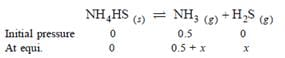Total pressure = 0.5 + 2x = 0.84 .. x = 0.17 atm.
Kp =PNH3 x PH2s = (0.5 + 0.17)(0.17)=0.11 atm2.

Test: Previous Year Questions: Chemical Equilibrium - Question 12

Phosphorus pentachloride dissociates as follows, is a closed reaction vessel,

PCl5(g) PCl3(g) + Cl2(g)

If total pressure at equilibrium of the reaction mixture is P and degree of dissociation of PCl5 is x, the partial pressure of PCl3 will be

[AIEEE 2006]

Detailed Solution for Test: Previous Year Questions: Chemical Equilibrium - Question 12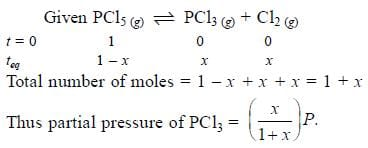Test: Previous Year Questions: Chemical Equilibrium - Question 13

The equilibrium constant for the reaction

SO3(g) SO2(g) + 1/2 O2(g) is Kc = 4.9×10-2. The value of Kc for the reaction

2SO2(g)+O2(g) 2SO3(g) will be

[AIEEE 2006]

Detailed Solution for Test: Previous Year Questions: Chemical Equilibrium - Question 13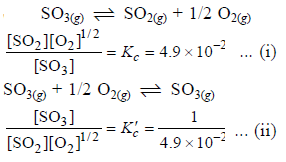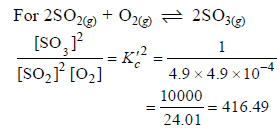Test: Previous Year Questions: Chemical Equilibrium - Question 14

For the following three reactions a, b and c, equilibrium constants are given

(a) CO(g) + H2O(g) CO2(g) +H2 (g); K1

(b) CH4(g) + H2O(g) CO(g) + 3H2(g); K2

(c) CH4(g) + 2H2O(g) CO2(g) + 4H2(g); K3

Which of the following relations is correct

Detailed Solution for Test: Previous Year Questions: Chemical Equilibrium - Question 14

Equation (c) = equation (a) + equation (b) Thus K3 = K1.K2

Test: Previous Year Questions: Chemical Equilibrium - Question 15

The equilibrium constants Kp1 and Kp2 for the reactions X  2Y and Z P + Q, respectively are in the ratio of 1 : 9. If the degree of dissociation of X and Z be equal then the ratio of total pressures at these equilibria is -

[AIEEE 2008]

Detailed Solution for Test: Previous Year Questions: Chemical Equilibrium - Question 15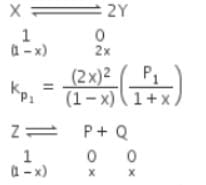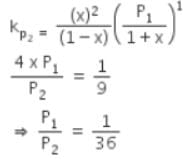Test: Previous Year Questions: Chemical Equilibrium - Question 16

A vessel at 1000 K contains CO2 with a pressure of 0.5 atm. Some of the CO2 is converted into CO on the addition of graphite. If the total pressure at equilibrium is 0.8 atm, the value of Kp is

[AIEEE 2011]

Detailed Solution for Test: Previous Year Questions: Chemical Equilibrium - Question 16

Kp depends upon the partial pressure of reactants and products so first calculate their partial pressure and then, calculate Kp,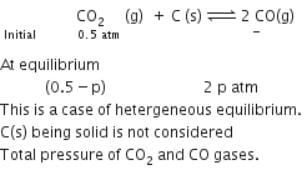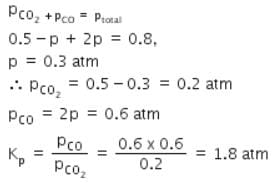Test: Previous Year Questions: Chemical Equilibrium - Question 17

The equilibrium constant (Kc) for the reaction N2(g)+O2(g) → 2NO(g) at temperature T is

4×10-4. The value of Kc for the reaction,
NO(g)→ ½ N2(g) + ½O2(g) at the same temperature is:

[AIEEE 2012]

Detailed Solution for Test: Previous Year Questions: Chemical Equilibrium - Question 17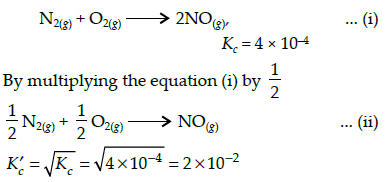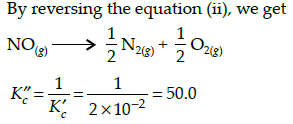## Chemistry Class 11

204 videos|331 docs|229 tests
 Use Code STAYHOME200 and get INR 200 additional OFF Use Coupon Code
Information about Test: Previous Year Questions: Chemical Equilibrium Page
In this test you can find the Exam questions for Test: Previous Year Questions: Chemical Equilibrium solved & explained in the simplest way possible. Besides giving Questions and answers for Test: Previous Year Questions: Chemical Equilibrium, EduRev gives you an ample number of Online tests for practice

## Chemistry Class 11

204 videos|331 docs|229 tests

### How to Prepare for NEET

Read our guide to prepare for NEET which is created by Toppers & the best Teachers